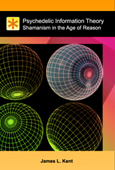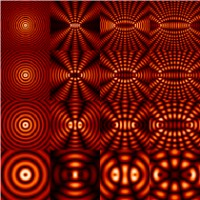Psychedelic Information Theory

Shamanism in the Age of Reason

References

Interference (wave propagation)

WikiPedia.org; Internet Reference, 2010.Figure 1 : In physics, interference is the addition (superposition) of two or more waves that results in a new wave pattern. Interference usually refers to the interaction of waves that are correlated or coherent with each other.

In physics, interference is the addition (superposition) of two or more waves that results in a new wave pattern. Interference usually refers to the interaction of waves that are correlated or coherent with each other, either because they come from the same source or because they have the same or nearly the same frequency. Interference in physics correponds to what in wireless communications is called multi-path propagation and fading, while the term interference has a different meaning in wireless communications.

Two non-monochromatic waves are only fully coherent with each other if they both have exactly the same range of wavelengths and the same phase differences at each of the constituent wavelengths.

The total phase difference is derived from the sum of both the path difference and the initial phase difference (if the waves are generated from two or more different sources). It can then be concluded whether the waves reaching a point are in phase (constructive interference) or out of phase (destructive interference).

Web Resource: en.wikipedia.org

Keywords: interference patterns, wave interference

 This reference is included in the following Listings: